Home > INT3 > Chapter 7 > Lesson 7.2.3 > Problem7-80

7-80.
1. Consider the triangle below. Homework Help ✎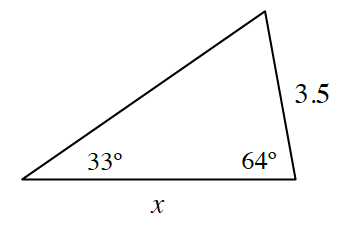1. Solve for x in the diagram.

2. What is the area of the triangle?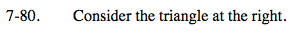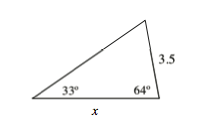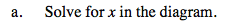Once you have determined the measure of the missing angle, use the Law of Sines.

$x \approx 6.4 \text{ units}$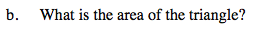Draw in a height, which forms two right triangles, then use trigonometry to find the height of the triangle.

$\approx \ 10.1 \text{ square units}$